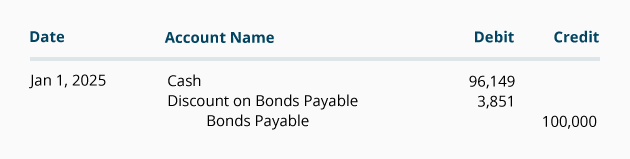# Calculating the Present Value of a 9% Bond in a 10% Market

Let's assume that a 9% \$100,000 bond is prepared in December 2021. By the time the bond is offered to investors on January 1, 2022 the market interest rate has increased to 10%. The date of the bond is January 1, 2022 and it matures on December 31, 2026. The bond will pay interest of \$4,500 (9% x \$100,000 x 6/12 of a year) on each June 30 and December 31.

To calculate the approximate price that an investor will pay for the corporation's bond on January 1, 2022, we need to calculate the bond's present value. The present value of the bond is the total of:

1. The present value of the bond's interest payments that will occur every six months, PLUS
2. The present value of the principal amount that occurs when the bond matures.

We calculate these two present values by discounting the future cash amounts by the market interest rate per semiannual period.

### 1. Present Value of the Bond's Interest Payments

The first step in calculating the bond's present value is to calculate the present value of the bond's interest payments. The interest payments form an ordinary annuity consisting of 10 payments of \$4,500 occurring at the end of each six month period as shown in the following timeline: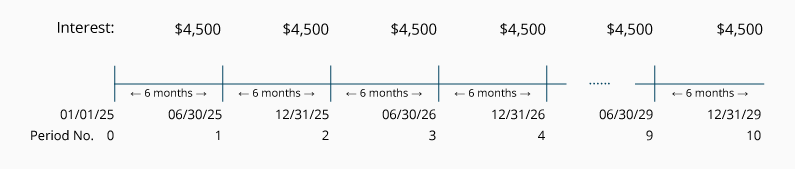To obtain the present value of the interest payments you must discount them by the market interest rate per semiannual period. In our example, the market interest rate is 5% per semiannual period. The 5% market interest rate per semiannual period is symbolized by i. (The market rate of 10% per year was divided by 2 semiannual periods per year to arrive at the market interest rate of 5% per semiannual period.)

The bond's life of 5 years is multiplied by 2 to arrive at 10 semiannual periods. The number of semiannual periods is symbolized by n.

Each semiannual interest payment of \$4,500 (\$100,000 x 9% x 6/12) occurring at the end of each of the 10 semiannual periods is represented by "PMT".

We use the above amounts (i = 5%, n = 10, PMT = \$4,500) in the following equation for calculating the present value of the ordinary annuity (PVOA):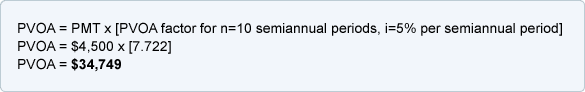(You will find more information about discounting an ordinary annuity at AccountingCoach.com's Explanation of Present Value of an Ordinary Annuity.)

Recall that this calculation determines the present value of the stream of interest payments only. The present value of the maturity amount will be calculated next.

### 2. Present Value of the Bond's Maturity Amount

The second step in calculating the present value of a bond is to calculate the present value of the maturity amount of the bond as shown in the following timeline: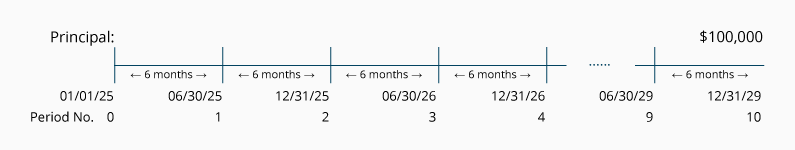Since the corporation's payment of the maturity amount occurs on a single date, we need to use the factors from a Present Value of 1 Table (PV of 1 Table). When using the PV of 1 Table we use the same number of periods and the same market interest rate that was used to discount the semiannual interest payments. In this case we use n = 10 semiannual periods, i = 5% per semiannual period, and the future value, FV = \$100,000.

Using the PV of 1 table, we see that the present value factor for n = 10, and i = 5% is 0.614.

The calculation of the present value (PV) of the single maturity amount (FV) is: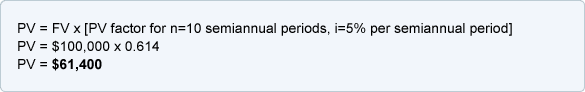### Combining the Present Value of a Bond's Interest and Maturity Amounts

Recall that the present value of a bond =

1. The present value of a bond's interest payments, PLUS
2. The present value of a bond's maturity amount.

The present value of the 9% 5-year bond that is sold in a 10% market is \$96,149 consisting of:

1. \$34,749 of present value for the interest payments, PLUS
2. \$61,400 of present value for the maturity amount.

The bond's total present value of \$96,149 is approximately the bond's market value and issue price.

It is reasonable that a bond promising to pay 9% interest will sell for less than its face value when the market is expecting to earn 10% interest. In other words, the 9% \$100,000 bond will be paying \$500 less semiannually than the bond market is expecting (\$4,500 vs. \$5,000). Since investors will be receiving \$500 less every six months than the market is requiring, the investors will not pay the full \$100,000 of a bond's face value. The \$3,851 (\$96,149 present value vs. \$100,000 face value) is referred to as Discount on Bonds Payable, Bond Discount, Unamortized Bond Discount, or Discount.

The journal entry to record the \$100,000 bond that is issued on January 1, 2022 for \$96,149 and no accrued interest is: